# Complementary Angles

The definition of complementary angles is two angles whose sum is 90°. Simply put, if you can add the measures of two angles together and the sum is equal 90° then the angles are complementary.

We are going to look at two types of complementary angles: adjacent and non-adjacent.

Adjacent: the angles share a common side and vertex and are "side-by-side".

Example 1:We have divided the right angle into 2 angles that are "adjacent" to each other creating a pair of adjacent, complementary angles.

Example 2: 60°+30° = 90° complementary and adjacent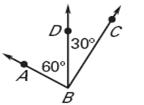Example 3: 50°+40° = 90° complementary and non-adjacent (the angles do not share a common side).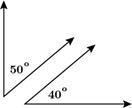Example 4: Given m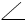1 = 43° and the m2 = 47° determine if the two angles are complementary.

43° + 47° = 90° therefore they are complementary.

Geometry & Algebra: solve for x then find mABD and mDBC.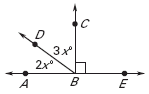ABD andDBC form a right angle therefore, they are complementary and their sum is 90°.

Write an equation 2x + 3x = 90
Combine like terms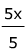=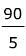Divide both sides by 5
x = 18°
mABD = 2x

Substitute 18 for x mABD = 2(18°) = 36°

mDBC = 3x

Substitute 18 for x mDBC = 3(18°) = 54°

A quick summary. if m1 + m2 = 90° then the angles are complementary. The angle pair can either be adjacent (side- by side) or non-adjacent.